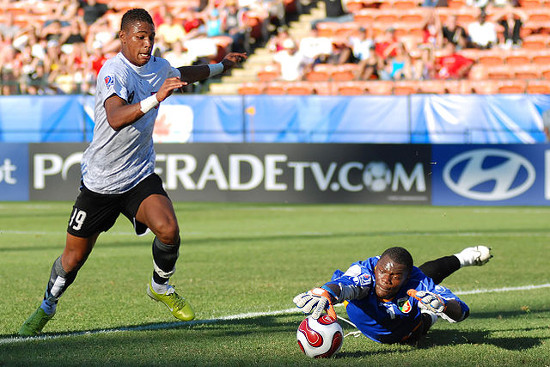# The Paradox of GoalsImage: Wikimedia Commons

Suppose that two teams of equal ability are playing football. If goals are scored at regular intervals, it seems natural to expect that each team will be in the lead for half the playing time. Surprisingly, this isn’t so: If a total of n = 20 goals are scored, then the probability that Team A leads after the first 10 goals and Team B leads after the second 10 goals is only 6 percent, while the probability that one team leads throughout the entire game is about 35 percent. (When the scores are equal, the leading team is considered to be the one that was leading before the last goal.) And the chance that one team leads throughout the second half is 50 percent, no matter how large n is.

Such questions began with a study of ballot problems: In 1887 Joseph Bertrand found that if in an election Candidate P scores p votes and Candidate Q scores q votes, where p > q, then the probability that P leads throughout the voting is (pq)/(p + q).

But pursuing them has led to “conclusions that play havoc with our intuition,” writes Princeton mathematician William Feller. If Peter and Paul toss a coin 20,000 times, we tend to think that each will lead about half the time. But in fact it is 88 times more probable that Peter leads in all 20,000 trials than that each player leads in 10,000 trials. No matter how long the series of coin tosses runs, the most probable number of changes of lead is zero.

“In short, if a modern educator or psychologist were to describe the long-run case histories of individual coin-tossing games, he would classify the majority of coins as maladjusted,” Feller writes. “If many coins are tossed n times each, a surprisingly large proportion of them will leave one player in the lead almost all the time; and in very few cases will the lead change sides and fluctuate in the manner that is generally expected of a well-behaved coin.”

(Gábor J. Székely, Paradoxes in Probability Theory and Mathematical Statistics, 2001; William Feller, An Introduction to Probability Theory and Its Applications, 1957.)